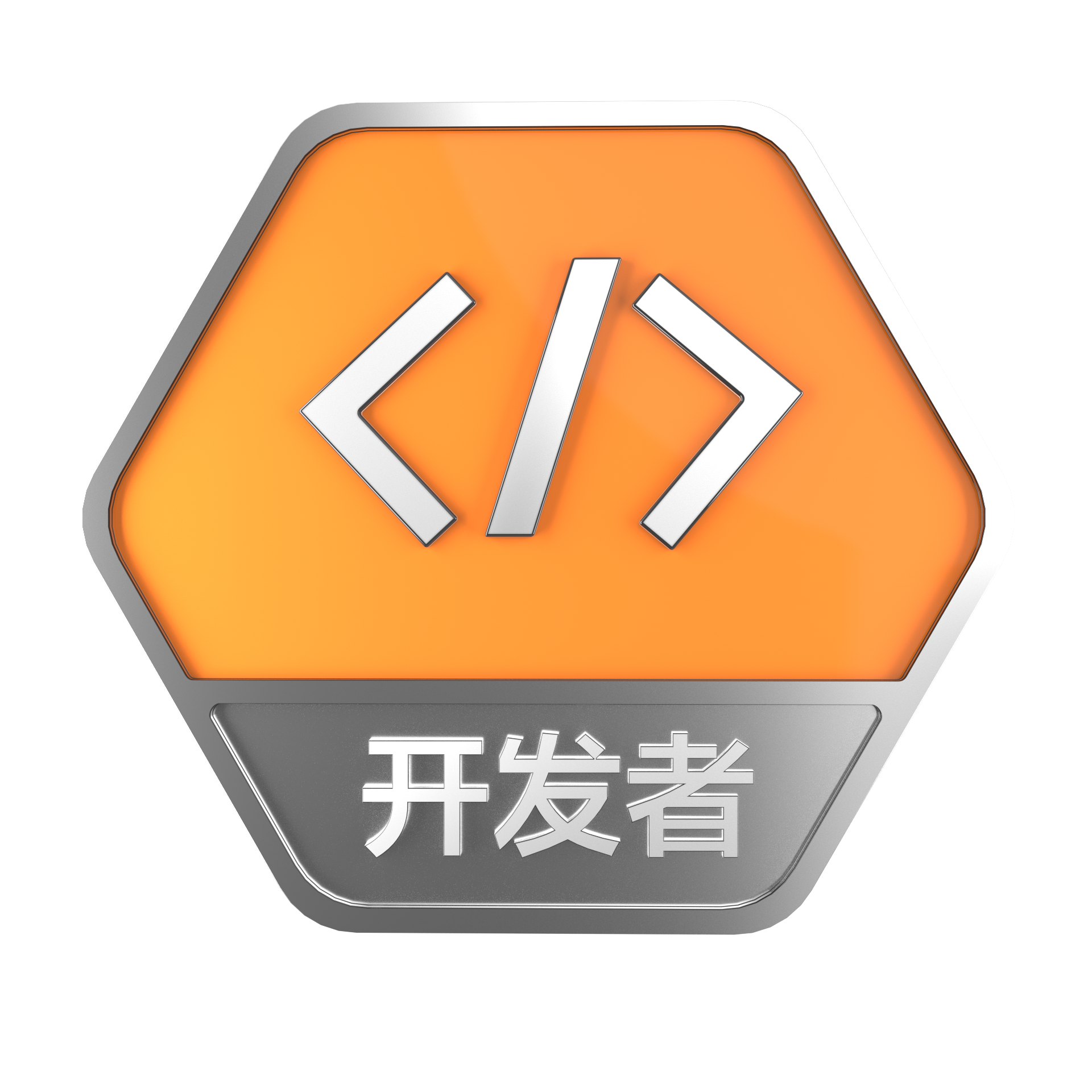Python学习笔记

+关注继续查看

Python学习笔记

1、整数 2、浮点数 3、字符串 4、布尔值 5、空值

The quick brown fox jumps over the lazy dog

print '\"To be, or not to be\": that is the question.\nWhether it\'s nobler in the mind to suffer.'

print r'''"To be, or not to be": that is the question.Whether it's nobler in the mind to suffer.'''

# -*- coding: utf-8 -*-      print u'中文'

>>> L = ['Adam', 'Lisa', 'Bart']

>>> L.append('Paul')

>>> print L

2insert（）方法，可以最近到指定地点

>>> L = ['Adam', 'Lisa', 'Bart']

>>> L.insert(0, 'Paul')

>>> print L

>>> L = ['Adam', 'Lisa', 'Bart', 'Paul']

>>> L.pop()

'Paul'

>>> print L

2>>> L = ['Adam', 'Lisa', 'Paul', 'Bart']

>>> L.pop(2)

'Paul'

>>> print L

>>> L = 'Paul'

>>> print L

tuple list 非常类似，但是，tuple一旦创建完毕，就不能修改了。

list['A', 'B']list作为一个整体是tuple的第3个元素。list对象可以通过 t 拿到：>>> L = t

Python代码的缩进非常严格，具有相同缩进的代码被视为代码块，

if 语句后接表达式，然后用:表示代码块开始。

age = 20

if age >= 18:

print 'END'

If-else语句

if age >= 18:

else:

print 'teenager'

If-elif-else语句

score = 85

if score>=90:

print 'excellent'

elif score>=80:

print 'good'

elif score>=60:

print 'passed'

else:

print 'failed'

L = [75, 92, 59, 68]

sum = 0.0

for score in L:

sum = sum + score

print sum / 4

for 循环不同的另一种循环是 while 循环，while 循环不会迭代 list tuple 的元素，而是根据表达式判断循环是否结束。

sum = 0

x = 1

while x < 100:

sum = sum + x

x = x + 2

print sum

for 循环或者 while 循环时，如果要在循环体内直接退出循环，可以使用 break 句。

sum = 0

x = 1

n = 1

while True:

sum = sum + x

x = x * 2

n = n + 1

if n > 20:

break

print sum

sum = 0

x = 0

while True:

x = x + 1

if x > 100:

break

if x % 2 == 0:

continue

sum = sum + x

print sum

100以内的两位数，请使用一个两重循环打印出所有十位数数字比个位数数字小的数，例如，232 < 3

for x in [1, 2, 3, 4, 5, 6, 7, 8, 9]:

for y in [0, 1, 2, 3, 4, 5, 6, 7, 8, 9]:

if x < y:

print x * 10 + y

d = {

'Lisa': 85,

'Bart': 59

}

1if 'Paul' in d:

print d['Paul']  通过key访问，返回value

2、通过dict本身提供的get方法

>>> print d.get('Bart')

59

>>> print d.get('Paul')

None          没有key值时，返回None

1、查找速度快

2、存储的key-value序对是没有顺序的

d = {

'Lisa': 85,

'Bart': 59

}

>>> print d

{'Lisa': 85, 'Adam': 95, 'Bart': 59}

3、作为 key 的元素必须不可变

Python的基本类型如字符串、整数、浮点数都是不可变的，都可以作为 key。但是list是可变的，就不能作为 key

1、key值不同，新添加，用赋值语句：>>> d['Paul'] = 72

2、如果key值已有，则更新，覆盖原有

d = {

'Lisa': 85,

'Bart': 59

}

for key in d:

print key + ':', d.get(key)

dict的作用是建立一组 key 和一组 value 的映射关系，dictkey是不能重复的。

set 持有一系列元素，这一点和 list 很像，但是set的元素没有重复，而且是无序的，这点和 dict key很像。

>>> s = set(['Adam', 'Lisa', 'Bart', 'Paul'])

>>> 'Bart' in s

True

>>> 'Bill' in s   大小写敏感

False

set的内部结构和dict很像，唯一区别是不存储value，因此，判断一个元素是否在set中速度很快。

set存储的元素和dictkey类似，必须是不变对象，因此，任何可变对象是不能放入set中的。

months = set(['Jan','Feb','Mar','Apr','Jun','Jul','Aug','Sep','Oct','Nov','Dec'])

x1 = 'Feb'

x2 = 'Sun'

if x1 in months:

print 'x1: ok'

else:

print 'x1: error'

if x2 in months:

print 'x2: ok'

else:

print 'x2: error'

s = set([('Adam', 95), ('Lisa', 85), ('Bart', 59)])

for x in s:

print x + ':', x

Lisa: 85

Bart: 59

1、添加新的元素

>>> s = set([1, 2, 3])

>>> print s

set([1, 2, 3, 4])

2、删除set中的元素      如果删除的元素不存在set中，remove()会报错：

>>> s = set([1, 2, 3, 4])

>>> s.remove(4)

>>> print s

set([1, 2, 3])

int()函数可以把其他数据类型转换为整数

str()函数把其他类型转换成 str

sum()函数接受一个list作为参数，并返回list所有元素之和。请计算 1*1 + 2*2 + 3*3 + ... + 100*100

L = []

x = 1

while x <= 100:

L.append(x * x)

x = x + 1

print sum(L)

Python中，定义一个函数要使用 def 语句，依次写出函数名、括号、括号中的参数和冒号:，然后，在缩进块中编写函数体，函数的返回值用 return 语句返回。

def square_of_sum(L):

sum = 0

for x in L:

sum = sum + x * x

return sum

print square_of_sum([1, 2, 3, 4, 5])

print square_of_sum([-5, 0, 5, 15, 25])

print打印返回结果，原来返回值是一个tuple

import math

t = math.sqrt(b * b - 4 * a * c)

return (-b + t) / (2 * a),( -b - t )/ (2 * a)

move(n, a, b, c)

A --> B

A --> C

def move(n, a, b, c):

if n == 1:

print a,'-->',c

return

move(n-1,a,c,b)

print a,'-->',c

move(n-1,b,a,c)

move(4, 'A', 'B', 'C')

B --> C

int()函数的第二个参数是转换进制，如果不传，默认是十进制 (base=10)，如果传了，就用传入的参数。

# OK:

def fn1(a, b=1, c=2):

pass

# Error:

def fn2(a=1, b):

Pass

def greet(name='world'):

print 'Hello,' + name + '.'

greet()

greet('Bart')

Hello,world.

Hello,Bart.

def average(*args):

sum = 0.0

if len(args) == 0:

return sum

for x in args:

sum = sum + x

return sum / len(args)

print average()

print average(1, 2)

print average(1, 2, 2, 3, 4)

>>> L[0:3]

>>> L[:]

1. 10个数；

2. 3的倍数；

3. 不大于505的倍数。

L = range(1, 101)

print L[:10]

print L[2::3]

print L[4:50:5]

5的倍数可以用 ::5 取出，要确定起始索引。

L = range(1, 101)

print L[-10:]

print L[-46::5]

def firstCharUpper(s):

return s.upper() + s[1:]

print firstCharUpper('hello')

print firstCharUpper('sunday')

print firstCharUpper('september')

Hello

Sunday

September

1. 有序集合：listtuplestrunicode

2. 无序集合：set

3. 无序集合并且具有 key-value 对：dict

for i in range(1,101):

if i % 7 == 0:

print i9473 0python学习笔记
918 0Python 学习笔记 - 装饰器
934 010839 0python 学习笔记（09）
849 013155 0【Python学习】Python解决汉诺塔问题

2977 0Python学习资源整理

1240 01747 0python 学习笔记（01）
578 0+关注
kaliarch
Devops,python,go
52

4

《2021云上架构与运维峰会演讲合集》

《零基础CSS入门教程》

《零基础HTML入门教程》